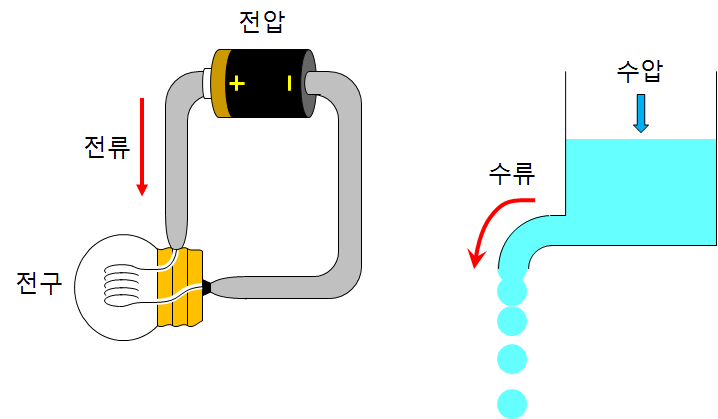# Ohm’s Law

## Ohm’s Law

When you connect the light bulb and the battery with a wire, the bulb lights up. This is because free electrons with negative charges (-) move along the wire, and this flow of charges is called current.
It is easy to understand if the current is compared to the flow of water. We can compare the electric current to the amount of water flowing per hour. For water to flow, a pressing pressure must be applied somewhere, compared to a voltage. Any property that impedes the flow of water becomes a resistance.The intensity of the current flowing through the circuit is proportional to the voltage and inversely proportional to the resistance. Ohm’s law is the relationship between voltage V, resistance R, and current I.

\begin{align} Current\; &∝\; \frac { Voltage }{ Resistance } \\ I\; &∝\; \frac { V }{ R } \end{align}

## The analogy of Ohm’s law

Consider the case of squeezing toothpaste. The force that presses the toothpaste is the voltage, the narrow hole of the toothpaste is the resistance, and the amount of toothpaste is thought of as the current.If you press the toothpaste tube strongly, toothpaste will come out well. ($$Current ∝ Voltage$$)
The narrower the hole, the less the toothpaste will come out. ($$Current\; \propto \; \frac { 1 }{ Resistance }$$)

## Electric shock

Electric shock is caused by current flowing in the body. If there is a voltage difference between one part of the body and the other part, an electric shock will occur.
The current has the property of passing through a place where it is easy to flow (where the electric resistance is small). Normally, there is a large resistance between the ground and the legs, so there is a very faint current flowing. However, if you are wet, your body’s electrical resistance will be small, allowing a large current to flow. Never touch the electric appliance while bathing.

• 0.001 A ~ You can feel the flow of current in your body.
• 0.005 A ~ It is painful.
• 0.01 A ~ It causes muscle contraction.
• 0.015 A ~ Muscles will be paralyzed.
• 0.07 A ~ It affects the heart. Larger currents are fatal. (Conceptual physics 7th Edition – Paul G. Hewitt)